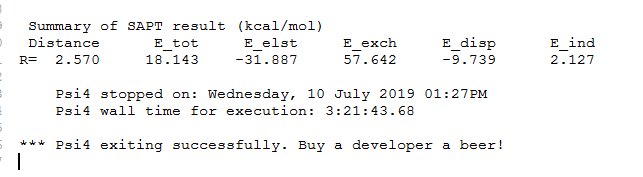# The total binding energy in SAPT energy decomposition turns out to be positive

I make an energy decomposition analysis in the complex LaCl3H2O. The structure has been optimized. By performing the SAPT calulation, I want to evaluate intermolecular interaction energy between LaCl3 and H2O. The input file is shown below,
memory 200000 MB

## molecule dimer { 0 1 La -1.245901 -0.030094 0.049950 Cl -2.495563 2.194252 -0.517413 Cl 1.386678 -0.062614 0.280512 Cl -2.525909 -2.306411 -0.142389

0 1
O -0.908811 -0.341351 2.578561
H 0.039773 -0.403213 2.763659
H -1.365765 -0.963412 3.157789
}
dimerdist= 2.569900

set {
basis def2-qzvpp-ri
scf_type DF
freeze_core True
}

energy(‘sapt2+(3)dmp2’)
E_disp = get_variable(‘SAPT DISP ENERGY’) * psi_hartree2kcalmol
E_elst = get_variable(‘SAPT ELST ENERGY’) * psi_hartree2kcalmol
E_exch = get_variable(‘SAPT EXCH ENERGY’) * psi_hartree2kcalmol
E_ind = get_variable(‘SAPT IND ENERGY’) * psi_hartree2kcalmol
E_tot = get_variable(‘SAPT TOTAL ENERGY’) * psi_hartree2kcalmol

psi4.print_out("\n")
psi4.print_out(" Summary of SAPT result (kcal/mol)\n")
psi4.print_out(" Distance E_tot E_elst E_exch E_disp E_ind\n")
psi4.print_out("%s %6.3f %10.3f %10.3f %10.3f %10.3f %10.3f\n" % (“R=”,dimerdist,E_tot,E_elst,E_exch,E_disp,E_ind))

However, after calculation, it is found that the total binding energy is positive, as shown in the picture attachment. Is it because of the basis set used for La?
I want to know what is the reason and appreciate it If anyone knows how to solve it. Thanks!You need to use standard orbital basis sets! The auxiliary basis sets (-RI/-JKFIT) will be added automatically. If you use them as main orbital basis sets many problems will arise.

Try
`basis def2-QZVPP`

Thanks for your reply. That is so kind of you! I have tried def2-qzvpp before. But an error is encountered, as shown in below,

Do you know the reason? Please grant instruction!

Do you have enough space in the your scratch directory? Did you specify one? The default (for Linux) is `/tmp` and the can have limits.